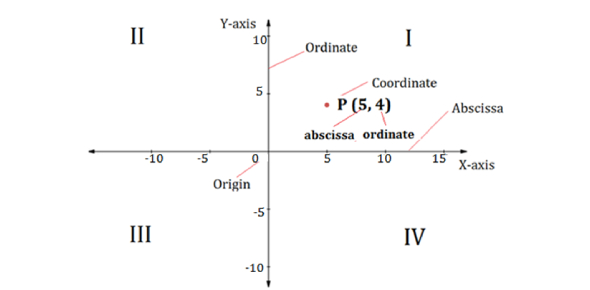# JEE Main: Co-ordinate Geometry Exam Quiz!

10 Questions | Attempts: 101
ShareSettings.

• 1.
If the line x = 1/2, is the directrix of a parabola y2 – kx + 6 = 0, then which of the following is a value of ‘k’?
• A.

– 4

• B.

– 6

• C.

– 1/4

• D.

– 8

• 2.
Equations x = t2+t+1 and y = t2 – t +1, represents which of the following curve?
• A.

An ellipse

• B.

A pair of straight

• C.

A hyperbola

• D.

A parabola

• 3.
Angle between tangents drawn from the point (2, 1) to the parabola y2 = – 32x is:
• A.
• B.
• C.
• D.
• 4.
A parabola has the origin at it focus and the line x = 2 as its directrix. Then the vertex of the parabola will be at:
• A.

(2, 0)

• B.

(0, 2)

• C.

(1, 0)

• D.

(0, 1)

• 5.
The eccentricity of the ellipse with its centre at the origin is 1/2. If one of the directrix is x = 4, then which of the following correctly represent the equation of ellipse.
• A.
• B.
• C.
• D.
• 6.
The number of values of c such that the straight line y = 4x + c touches the curve ;is:
• A.

4

• B.

0

• C.

Infinite

• D.

2

• 7.
Tangents are drawn to the ellipse at the end of the latus retum. The area of the quadrilateral so formed is:
• A.

54

• B.

27

• C.

27/2

• D.

36

• 8.
If x = 9 is the chord of the contact of the hyperbola x2 – y2 = 9, then the equation of the corresponding pair of tangents is
• A.
• B.
• C.
• D.
• 9.
2x + √6 y = 2 touches the hyperbola x2 – 2y2 = 4, then the point of contact is:
• A.
• B.
• C.

Both a & b

• D.

None of these

• 10.
The eccentricity of hyperbola 3x2 – y2 = 4 is:
• A.

1

• B.

5

• C.

– 2

• D.

2

## Related TopicsBack to top
×

Wait!
Here's an interesting quiz for you.